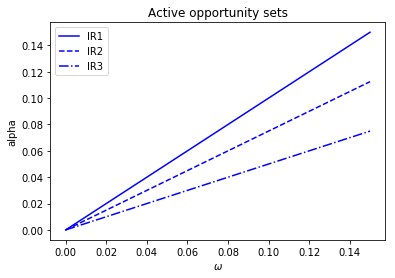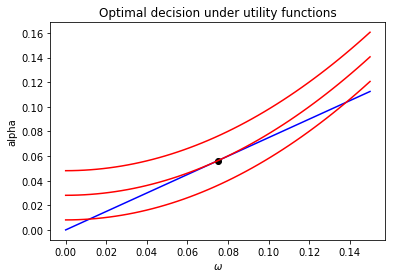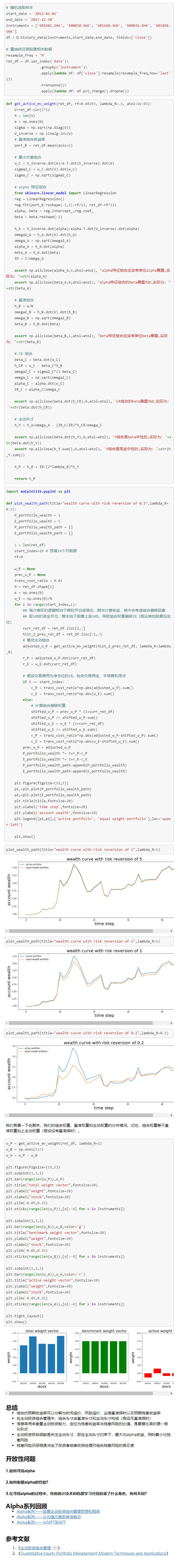• 主动投资组合管理】第1章 绪论
2021-02-03 11:58:33

# 1. 绪论

## 1.2 战略性概述

• 把风险预测问题与收益预测问题分离，关心主动奉献和主动收益率

### 信息率

• 残差收益率：资产收益率与基准收益率不相关的部分
• 信息率IR（information ratio）=残差收益率年化预期值/年化波动率
• 信息率定义了主动投资经理可选的投资机会的集合
• 信息率越高，主动管理的空间越大
• 信息率来源：IC与BR
• IC（信息系数，information coefficient）：收益率的预测值和实现值之间的相关系数
• 二者关系： I R = I C ∗ B R IR=IC*\sqrt{BR}

### 风险调整预期收益率

• 残差收益率-残差风险的二次惩罚项
• 可以用无差异曲线来描述偏好。附加值相同，偏好无差异。
• 零残差风险组合对应的残差收益率被成为“确定性等价”残差收益率。
• 主动管理可获得的最高附加值正比于信息率的平方。

主动管理组合五大基本元素：收益、风险、基准、偏好和IR。加主动管理实践的元素：与一致预测不同的预期收益率预测

主动管理即预测。

### 预测基本定律：

a l p h a = 波 动 率 ∗ I C ∗ 标 准 分 值 alpha=波动率*IC*标准分值

• 标准分值即Z值。alpha即残差收益率的预测值。波动率为残差波动率

## 1.3 参考文献

更多相关内容
• 许多机构投资者关注主动风险和残差风险甚于总风险 主动风险主要依赖于主动头寸的规模，而不是基准头寸的规模 风险造成的成本与方差成正比 风险模型识别重要的风险来源，并把风险分解为多个组成部分 2. 定义风险 ...

# 1. 导言

要点

• 风险是收益率的标准差
• 风险不具有可加性
• 许多机构投资者关注主动风险和残差风险甚于总风险
• 主动风险主要依赖于主动头寸的规模，而不是基准头寸的规模
• 风险造成的成本与方差成正比
• 风险模型识别重要的风险来源，并把风险分解为多个组成部分

# 2. 定义风险

所有的风险定义本质上都依赖于收益率的概率分布。标准差衡量了收益率在均值附近分布范围的宽度，也称波动率（Volatility）。

有批评指出，投资者认为较低的或负的收益率才是风险，因此诞生了半方差（semivariance，低于均值的收益率样本）和下行风险（downside risk，定义不统一）的定义以及其变体目标半方差（target semivariance，低于目标收益率的样本）。

• 下行风险本身问题：
• 定义和统计属性不明确
• 难以将但只资产汇总到组合，导致大规模投资组合难以计算
• 若收益率对称，downside risk往往是标准差的特定倍数，不蕴含额外信息。若不对称，则收益率的对称性不稳定，难以预测downside risk。
• 仅用到一半样本，损失统计精度
• 更关注尾部样本。

损失概率（shortfall probability，收益率落在目标值以下的概率）与下行风险存在的问题相似。在险价值（value at risk，先确定目标损失值，再计算收益率低于该值概率）与损失概率概念相似。

以上风险定义方法的前提条件为收益率正态分布假设。

# 3. 标准差

σ p = ( w 1 σ 1 ) 2 + ( w 2 σ 2 ) 2 + 2 w 1 w 2 σ 1 σ 2 ρ 1 , 2 \sigma_p=\sqrt{(w_1\sigma_1)^2+(w_2\sigma_2)^2+2w_1w_2\sigma_1\sigma_2\rho_{1,2}}

上式说明投资组合整体风险小雨部分风险只和，这是投资组合分散化的关键。

如果一个等权重组合，每个资产风险都为 σ \sigma ，且各个资产相关系数为0，则组合风险为:
σ p = σ N \sigma_p=\frac{\sigma}{N}
若以上组合各个资产相关系数均为 ρ \rho ，则组合风险为：
σ p = σ 1 + ρ ( N − 1 ) N \sigma_p=\sigma\sqrt{\frac{1+\rho(N-1)}{N}}
假设任意不重叠的两个时期收益率是不相关的（即收益率序列自相关性为0），则方差在时间维度上具有可加性，因此存在年度月度日度波动率之间的根号倍数关系。

主动收益率（active return）为组合超额收益率与基准收益率之差，记为 r p a = r p − r b r_{pa}=r_p-r_{b} 。主动风险（也称跟踪误差tracking error）为 r p a r_{pa} 的标准差：
ψ p = S t d ( r p a ) = S t d ( r p − r b ) \psi_p=Std(r_{pa})=Std(r_p-r_b)

主动风险取决于主动暴露和股票风险，不依赖该股票在基准组合中的权重。有时令大盘股有更高的风险暴露是出于交易成本和流动性的考量。

残差风险是与系统性收益率正交的收益率的风险。设组合 p p 关于组合 b b 的残差风险用 ω p \omega_p 表示，则
ω p = σ p 2 − β p 2 σ b 2 \omega_p=\sqrt{\sigma_p^2-\beta_p^2\sigma_b^2}
其中
β p = C o v ( r p , r b ) V a r ( r b ) \beta_p=\frac{Cov(r_p,r_b)}{Var(r_b)}
这里与CAPM又联系起来了。

# 4. 基本的风险模型

待更新…

展开全文• 在本文中，我们采用主动管理的基础和两基金分离的方法，利用投资者可以根据感知的自然状态在市场投资组合和无风险资产之间切换的事实。 我们的目的是通过使用我们自己的非常规多因素模型测试绩效来评估是否存在主动...
• Grinold和Kahn解决的主动投资组合管理（第二版）中的问题的解决方案。 可能有一些错误。 主要章节练习的解答：Nicholas J. Hestand。 技术附录中的练习解决方案：Nicholas J. Williams。 如果您最终赚了很多钱，...
• 第2章 一致预期收益率：资本资产定价模型 ...CAPM认为被动管理主动管理的低风险替代选择 CAPM为一致预期收益率提供了有效来源。主动基金经理的成功程度与其预测优于一致预测的程度相匹配 CAPM讨论的是预期收益

# 第2章 一致预期收益率：资本资产定价模型

## 2.1 导言

CAPM的重要衍生品是一套确定一致预期收益率的步骤。主动管理决策就是我们的预测与一致预期收益率之间的差异驱动的。

### 本章要点

1. 任何股票收益率都可以分为系统性（市场）和非系统性（残差）部分
2. CAPM要求残差收益率期望为0
3. CAPM容易理解，也相对容易实践
4. CAPM有一个关于市场有效的逻辑
5. CAPM认为被动管理是主动管理的低风险替代选择
6. CAPM为一致预期收益率提供了有效来源。主动基金经理的成功程度与其预测优于一致预测的程度相匹配
7. CAPM讨论的是预期收益率，而非风险

### 预测收益率方法问题

• 历史收益率替代法：1. 历史数据包含大量样本误差；2. 股票样本空间随时间变化
• 套利定价理论（arbitrage pricing theory, APT）：不能作为一致预期收益率来源

## 2. 2 收益率分解

考虑任意一个投资组合 P P ，组合 P P 的收益率为 r P r_P ，市场组合 M M 的超额收益率为 r M r_M ，超额收益率即市场组合收益率减无风险资产的收益率。定义组合的 β P \beta_P
β P = C o v ( r P , r M ) V a r ( r M ) \beta_P=\frac {Cov(r_P,r_M)}{Var(r_M)}
β P \beta_P 是对未来的预测值，但其观念和名称却来自历史数据的回归。用组合 P P 的超额收益序列 r P r_P 对市场超额收益率序列 r M r_M 回归。
r P ( t ) = α P + β P r M ( t ) + ϵ P ( t ) r_P(t)=\alpha_P+\beta_Pr_M(t)+\epsilon_P(t)
这一计算得到的 β P \beta_P α P \alpha_P 均称作历史的 β \beta α \alpha ，但可以作为未来 β \beta α \alpha 的合理预测。

基于以上思路，如果已知组合 β \beta ，可以将其超额收益分解为市场和残差两部分
r P = β P r M + θ P r_P=\beta_Pr_M+\theta_P
其中 θ P \theta_P 为残差收益率，与市场收益率 r M r_M 不相关。同时，可以将组合方差进行分解：
σ P 2 = β P 2 σ P 2 + ω P 2 \sigma^2_P=\beta^2_P\sigma^2_P+\omega^2_P

β \beta 使任意投资组合可以被分解为市场部分和残差部分，且两者不相关。目前CAPM并无前提假设。

## 2.3 CAPM

CAPM模型下，任何股票和投资组合的残差收益率为零，即 E ( θ P ) = 0 E(\theta_P)=0 ，因此预期超额收益率与股票的 β \beta 成正比。此处对于市场组合必然成立，但隐含了所有投资者预期均相同的假设，过于严格。

## 2.4 CAPM的合理性

CAPM逻辑：投资者承担的必要风险会得到补偿，非必要风险不会得到任何补偿。所有持有不同于市场组合的投资者，都在进行一个零和游戏。

## 2.5 CAPM和有效市场理论

有效市场假说

• 弱有效：技术分析无效
• 半强有效：技术分析+基本面分析无效（公开信息无效）
• 强有效：所有分析都无效

CAPM同样认为投资者不能战胜市场，因为所有持有非市场组合的投资者里一定有残差收益率为负的“更傻的傻瓜”，只要不存在这样的傻瓜，投资者就无法战胜市场组合。

## 2.7 后验与先验

关于预期：

根据CAPM可画出SML（security market line，证券市场线）：横坐标为所有股票的 β \beta ，纵坐标为对应年化收益率。斜率为市场组合超额收益率 r M r_M ，纵截距为无风险收益 i F i_F

关于后验：
不稳定市场线（insecurity），将股票实际收益率画入上述图中。当实际收益率点落在CAPM预测点下方，投资者取得超额收益。

## 2.9 CAPM效果如何

CAPM中，关于任何股票都不存在超额收益这一结论显然不符合常理。但经历了几百年的研究与实践，CAPM依然很难被推翻。

## 2.10 与主动投资经理的关联

收益率分解不需要任何理论，只需要预测 β \beta ，可以帮助投资经理控制风险。CAPM的思路可以避免市场择时的风险，并集中精力研究一致预期为0的残差收益率。

## 2.16 技术附录

风险定义为超额收益率的年华标准差。单一投资期。基础假设

1. 存在一个无风险资产
2. 所有一阶矩和二阶矩均存在
3. 不存在零风险的投资组合
4. 组合C（最小方差组合）预期超额收益率为正值

特征组合：代表某种属性的组合，唯一地定义资产的某种属性。通过计算一个资产与特征组合的协方差来确定组合对该属性的暴露值。

### 命题

命题1: 对任意一个属性 a ≠ 0 a\neq0 ，对 a a 具有单位暴露的所有组合中，存在唯一一个组合 h a h_a 使得风险最小，称为 a a 的属性组合
h a = V − 1 a a T V − 1 a h_a=\frac{V^{-1}a}{a^TV^{-1}a}

命题2：特征组合 h a h_a 的方差如下
σ a 2 = h a T V h a = 1 a T V − 1 a \sigma^2_a=h^T_aVh_a=\frac{1}{a^TV^{-1}a}
命题3：全体资产对组合h_a的 β \beta 等于 a a
a = V h a σ a 2 a=\frac{Vh_a}{\sigma^2_a}

命题4：对于两个属性 a a d d ，令 a d a_d d a d_a 分别为组合 h a h_a 对特征 a a 的暴露及组合 h d h_d 对特征 d d 的暴露，两个特征组合协方差满足
σ a , d = a d σ a 2 = d a σ a 2 \sigma_{a,d}=a_d\sigma^2_a=d_a\sigma^2_a

命题5：如果 K K 是一个正实数，则属性 K a Ka 的特征组合是 h a / K h_a/K 。特征组合对其属性只能具有单位暴露度。

命题6：如果特征 a a 是特征 d d f f 的加权线性组合，则 a a 的特征组合也是 d d f f 的加权线性组合
h a = ( K d σ a 2 σ d 2 ) h d + ( K f σ a 2 σ f 2 ) h f h_a=(\frac{K_d\sigma^2_a}{\sigma^2_d})h_d+(\frac{K_f\sigma^2_a}{\sigma^2_f})h_f
其中
1 σ a 2 = ( K d a d σ d 2 ) + ( K f a f σ f 2 ) \frac{1}{\sigma^2_a}=(\frac{K_da_d}{\sigma^2_d})+(\frac{K_fa_f}{\sigma^2_f})

展开全文• 用于教育目的或研究基础... 该模型应用现代投资组合管理原则来组合被动和主动投资组合组件。 该模型允许管理多头/空头头寸，并允许对估计参数进行模块化扩展。 该示例引用了 Bodie、Kane 和 Marcus 的《投资》第 9 版。matlab
• 这篇教程的主要目的是介绍主动投资组合管理的一些重要概念，帮助不熟悉这一领域的读者能够了解这个方法论的核心思想和价值。我们先介绍主动投资的概念，然后引入主动投资里最重要的概念——信息率。 接着我们分别从...

## 主动投资管理——信息率

前面文章为大家介绍了许多AI量化投资理念，这篇教程的主要目的是介绍主动投资组合管理的一些重要概念，帮助不熟悉这一领域的读者能够了解这个方法论的核心思想和价值。我们先介绍主动投资的概念，然后引入主动投资里最重要的概念——信息率。

接着我们分别从主动投资组合最优化框架，以及收益、风险的权衡展开，最后我们通过一个实际的主动投资组合的案例实验来结束讨论。

## 主动投资概念定义

之前我们已经介绍了经典的MPT，它的核心思想是市场是均衡的，不存在套利机会，投资者应该投资市场指数，从而分散股票的非特异风险并使得交易成本最低化。

但是，金融市场的从业人员、投资者一般都很难相信市场是完全有效的，甚至对于技术分析派的交易者，也会否认市场的弱势有效性。但是MPT确实有很多概念和工具值得我们借鉴，
于是，以Grinold为代表的主动投资理论便蓬勃发展起来，并且继承了MPT的诸多思想。

所谓主动投资是相对于被动投资而言的，假设有一家私募基金，投资者并且私募管理者以基金相对于沪深300指数的相对收益作为评估基金的标准，那么我们便认为沪深300指数期间的成分股权重作为这个资金投资组合的被动权重或者基准权重，
而基金任何偏离基准权重的情况都是基金的主动投资行为，因为基金管理者认为这些偏离能给组合带来相对于基准更高的风险调准后收益，也就是我们所谓的alpha收益或者风险调整后alpha。如果一个基金在整个投资期间没有任何主动权重，
那么它就是一只指数基金，因为它复制了指数组合，并且也获得了相同的指数收益。如果是一家对冲基金，它以绝对收益作为衡量自己业绩的标准，那么它的被动权重就是现金或者无风险资产，任何风险资产头寸都成为它的主动头寸。

一个基金管理者或者投资者采取主动投资，便是他认为他的主动行为上（如选股）相对于基准，能够提供额外的alpha收益，也就是说他相信自己在某些方面的投资能力能够创造alpha。主动投资组合管理便是一个系统的方法论和流程，
用以帮助相信具有alpha能力的投资者或者基金管理者来寻找和管理alpha。

## 主动投资组合

为简介易懂，我以下面的表格作为例子。我们的基准组合是一个包含四个股票的全额投资等权组合，在表格的第二列是我们的基准权重。然后在一个假设场景中，基金经理认为风险资产配置太高，于是降低到90%，无风险资产权重提升至10%。
基金经理根据自己的独特信息来源以及选股能力，给出了下一个投资期的股票预期收益。并基于预期收益信息，基金经理进一步调整了风险资产权重，对应的是表格中的基金权重，作为验证，我们可以计算得到基金权重的总和是100%。
在上面我们也说过了，主动投资的定义是于基准的偏离，也就是实际资产组合与基准组合的偏差，于是我们把基金权重减去基准权重得到了主动权重，在我们表格中的第三列。主动权重是相对偏离，所以它的权重是可以为负的，但是我们还
看到了一个有趣的现象，那就是主动权重的和为0，也就是说主动组合是一个现金中性组合。最后，我们还计算了组合的实际收益率为31.7%，基准为14.5%，也就是说我们实现了17%的alpha，或者说主动收益率。

基准组合基准权重主动权重基金权重预期收益期末收益期末资本收益
现金0%10%10%5%5%0.5%
股票125%-15%15%10%8%1.2%
股票225%15%60%50%40%24%
股票325%-10%-10%-20%-10%1%
股票425%0%25%15%20%5%
合计100%0%100%N/AN/A31.7%

## 预期收益率的构成

我们可以将预期收益率分解为四部分，分别是无风险收益（时间溢价）、业绩基准部分（风险溢价）、基准择时部分（超额业绩基准收益率）、alpha部分（预期残差收益率），即：

\begin{align*}
\mathbb{E}[r_i] = r_f + \beta_i \mu_b + \beta_i \Delta f_b + \alpha_i
\end{align*}

#### 时间溢价 r f r_f

时间溢价表示投资者放弃当前资产的投资转而投资无风险资产所获得的收益。称之为时间溢价代表对时间的补偿。一般我们可以采取银行间拆借利率作为无风险资产收益率，比如shibor。

#### 风险溢价 β i μ b \beta_i \mu_b

这里采用了CAPM的概念，基准收益 μ b \mu_b 一般指长期的基准超额收益。低beta资产具有较低的风险溢价，高beta具有较高的风险溢价。

#### 超常业绩基准收益率 β i Δ f b \beta_i \Delta f_b

如果你相信在未来相对短周期的投资期内（如下一季度、下一年），预期业绩基准收益率不同于 μ b \mu_b ，那么 Δ f b \Delta f_b 就衡量了业绩基准在临近的未来时期上的预期超额收益率与长期预期超额收益率的差，也就是我们常说的大盘择时。
而Grinold认为，大多数投资机构经理都不使用业绩基准择时，而忽略它也将大大降低学习主动管理的学习曲线，所以如无特别说明，我们下面以及以后的教程都会假设没有基准业绩择时，也就是 Δ f b = 0 \Delta f_b = 0 。我会在专门的教程里讨论基准择时。

#### Alpha

α i \alpha_i 即股票预期残差收益率，也就是我们最感兴趣的一部分，衡量投资者的投资能力，如预测能力、选股能力。

#### 主动收益率与残差收益率

残差收益率和风险为：

\begin{align*}
\theta_p &= r_p - \beta_p r_b\
\omega_p &= std(\theta_p)
\end{align*}

主动收益率和风险为:

\begin{align*}
r_{pa} &= r_p - r_b = \theta_p - \beta_{pa} r_b\
\psi_p &= std(r_{pa}) = \sqrt{\omega_p^2 + \beta_{pa}^2 \sigma_b^2}
\end{align*}

投资经理在避免基准择时的情况下，并设置 β p = 1 \beta_p=1 ，那么主动收益率就是残差收益率。如果投资经理进行基准择时，从上述公式可以看到，主动收益率等于残差收益率与业绩基准择时收益率的和。

## 信息率

这里，我们将介绍衡量主动投资成功的标准，那就是信息率。不过在谈信息率之前，我们先要谈谈alpha。

#### 定义alpha

向未来看，即先验alpha是对残差收益率的预测。向过去看，即后验alpha是实现的残差收益率的平均值。

alpha类似beta，是利用线性回归将组合收益率分解为与基准相关的部分（线性相关)，以及与基准不相关的残差部分（非线性相关）。于是：

\begin{align*}
r_{p}(t) &= \alpha_p + \beta_p r_b(t) + \epsilon_p(t)
\end{align*}

上面的 α p \alpha_p β p \beta_p 分别是我们的后验alpha与后验beta。马上，我们可以推导出组合的残差收益率：

\begin{align*}
\theta_{p}(t) &= \alpha_p + \epsilon_p(t)
\end{align*}

α p \alpha_p 便是平均残差收益率， ϵ p ( t ) \epsilon_p(t) 是残差收益率的随机项（均值为0）。

当我们向未来看，我们的先验alpha就是对残差收益率的预测。令 θ i \theta_i 表示股票i的残差收益率，于是有：

\begin{align*}
\alpha_i &= \mathbb{E}[\theta_i]
\end{align*}

组合alpha为：

\begin{align*}
\alpha_p &= \boldsymbol{w^{\prime} \alpha}
\end{align*}

根据定义，基准的残差收益率总是为0，无风险资产的残差收益率也是0，因此任何有业绩基准和现金构成的投资租着的alpha也必然为0，即被动投资的alpha为0。

#### 后验信息率——对业绩的衡量

信息率用IR来表示，定义为年化残差收益率与年化残差风险的比值:

\begin{align*}
IR_p = \frac{\bar{\theta}_p}{\omega_p} = \frac{\bar{\alpha_p}}{std(\alpha_p)}
\end{align*}

若不存在基准择时，那么 β p = 1 \beta_p=1

\begin{align*}
IR_p = \frac{\bar{\alpha_p}}{std(\alpha_p)} = \frac{r_p-r_b}{std(r_p-r_b)}
\end{align*}

上面这个公式我们是不是觉得有点眼熟，是的，很像夏普比率：

\begin{align*}
SR = \frac{r_p-r_f}{std(r_p)}
\end{align*}

SR代表单位总风险所蕴含的超额回报，IR在这个基础上，引入了相对性，它衡量了主动头寸组合的单位风险所蕴含的主动收益，换言之，即风险调整后的alpha。
如果我们将基准设为无风险资产，那么IR就是SR，之前我们也讨论过，SR用来衡量追求绝对收益的对冲基金更加合适，对于追求相对收益的投资基金或者投资策略，
就不那么合适了。

在《主动投资组合管理》，grinold给出了衡量IR的参考分布：

分位数信息率
101
250.5
500
75-0.5
90-1

Grinold认为整体而言，投资经理的费前信息率非常接近上表，是一个对称分布，所以主动管理是一个零和博弈游戏。在实践中，信息率高于1是卓越，高于0.5的信息率算是优秀了。

#### 残差前沿

在被动投资里，我们的投资机会集就是与有效前沿相切的CML，所有投资经理、投资者都一样。而在主动投资中，IR绝对了投资经理、投资者的能力水平，并且只有在相同的业绩基准下，投资经理的IR才有对比的合理性。
信息率为主动投资经理定义了一条“预算约束”，即残差前沿：

\begin{align*}
\alpha_p = IR \cdot \omega_p
\end{align*}

在最优的情形下，投资经理只能通过增加相应程度的残差风险来增加预期残差收益率。下面我们画出了主动投资的机会集，IR是图中的斜率，x/y轴分别代表残差收益率和残差风险。

import matplotlib.pyplot as plt
omegas = np.linspace(0, 0.15,50)
IR1,IR2,IR3 = [1,0.75,0.5]
alphas_1 = IR1*omegas
alphas_2 = IR2*omegas
alphas_3 = IR3*omegas
plt.plot(omegas, alphas_1, 'b-',label='IR1')
plt.plot(omegas, alphas_2, 'b--',label='IR2')
plt.plot(omegas, alphas_3, 'b-.',label='IR3')
plt.title("Active opportunity sets")
plt.xlabel("$\omega$")
plt.ylabel("alpha")
plt.legend()
plt.show()## 主动投资目标函数

首先，让我们重温下MPT里的最优组合问题阐述，其中f是超额收益：

\begin{align*}
Minimize \qquad & \boldsymbol{w^{\prime} f} - \lambda \boldsymbol{w^{\prime} \Sigma w}\
s.t \qquad & \boldsymbol{w^{\prime}} \boldsymbol{1} = 1
\end{align*}

在主动投资组合管理中，我们关注的目标不再是我们的组合头寸，而是主动头寸，还记得我们的组合头寸总是等于基准头寸加上主动头寸么？

那么，在没有基准择时的情况下，我们的问题便转化为寻找最优无主动beta、现金中性的主动头寸：

\begin{align*}
Maximize \qquad & \boldsymbol{w_a^{\prime} \alpha} - \lambda \boldsymbol{w_a^{\prime} (\Sigma - \beta \beta^{\prime} \sigma_b^2) w_a}
= \alpha_p - \lambda \omega_p^2\
s.t \qquad & \boldsymbol{w_a^{\prime}} \boldsymbol{1} = 0\
\qquad & \boldsymbol{\beta^{\prime} w_a} = 1
\end{align*}

#### 给定无差异曲线下的最优解

我们有两个函数，分别是无差异曲线族和残差前沿：

\begin{align*}
U &= \alpha - \lambda \omega^2, \quad \lambda \in \mathbb{R^{+}}\
\alpha & = IR \cdot \omega
\end{align*}

为了找到两条曲线的焦点，把残差前沿带入无差异效用曲线：

\begin{align*}
\lambda \omega^2 - IR \cdot \omega + U = 0
\end{align*}

因为相切，所以上述曲线只有一个交点，最终得到：

\begin{align*}
U^{\ast} &= \frac{IR^2}{4\lambda}\
\alpha^{\ast} &= \frac{IR^2}{2 \lambda}\
\omega^{\ast} &= \frac{IR}{2 \lambda}
\end{align*}

下面我们用python代码画出了无差异效用曲线和残差前沿，并且得到了最优组合。

omegas = np.linspace(0, 0.15,50)
IR = 0.75
lam = 5
alphas = IR*omegas
opt_va = IR**2/(4*lam)
opt_alpha = IR**2/(2*lam)
opt_omega = IR/(2*lam)

plt.plot(omegas, alphas, 'b-')
plt.plot(opt_omega, opt_alpha, 'ko')
# 无差异效用曲线
U = opt_va + lam * omegas**2
plt.plot(omegas, U, 'r-')
plt.plot(omegas, U+0.02, 'r-')
plt.plot(omegas, U-0.02, 'r-')
plt.title("Optimal decision under utility functions")
plt.xlabel("$\omega$")
plt.ylabel("alpha")
plt.show()#### 主动投资组合案例

下面我们用一个toy example作为这篇教程的结尾。这个案例实验取自于《Alpha系列——从均值方差到有效前沿》，我们随机选取了五个股票，并重抽样还原至月收益数据，选取时间范围为2012年处到2017年底，同时以等权投资组合作为我们的基准业绩组合。
无风险资产收益率我们去一年期chibor利率，回测区间为2014年到2017年底，预留两年的数据用以计算必要的参数。

下面我们给出了实际可运行的python代码，其中get_active_mv_weight函数用以计算主动投资下的组合权重，plot_wealth_path函数画出我们最终的净值曲线，我们分别设置对残差风险厌恶系数分别为5、1、0.2情况下的净值曲线。从图中我们可以很明显地
看到残差风险厌恶系数对我们组合地残差风险的影响（与基准变动程度的偏离），同时我们的净值曲线大多在基准上面，说明了我们的主动头寸贡献了alpha，回忆一下，我们组合中的基准头寸beta为1，alpha为0。#### 源码地址：《Alpha系列——主动投资管理之信息率》

本文由BigQuant人工智能量化投资平台原创推出，版权归BigQuant所有，转载请注明出处。

展开全文量化投资 量化策略
• 投资组合管理策略，绩效评价指标，选股能力和择时能力
• 清华编程高手尹成带你基于算法实践python量化交易量化交易是指以先进的数学模型替代人为的主观判断，利用计算机技术从庞大的历史数据中海选能带来超额收益的多种...两者的区别在于定量投资管理是“定性思想的量化...
• active portfolio management --A Quantitative Approach for Providing Superior Returns and Controlling Risk by Richard C. Grinold and Ronald N. Kahn.
• 在估值方面的区别：被动性投资策略的估值是对所跟踪的指数进行估值，主动性投资策略的估值是对所持仓的股票分别估值并加权，但是主动投资时往往仓位会根据市场行情经常做出减仓加仓和调仓换股的操作，这就加大了估值...
• 5. 投资组合构建模型 5.1 基于规则的投资组合构建模型 5.2 基于优化的投资组合构建模型 5.2.1 优化工具的输入变量 5.2.2 优化技术 6. 执行模型 6.1 订单执行算法 6.1.1 进取订单和被动订单 6.1.2 其他订单...量化交易 数据挖掘
• 4、《主动投资组合管理：创造高收益并控制风险的量化投资方法》 这本书详细讲解了主动投资管理的基本原则和理论，也提供了实践的细节。值得一提的是，这本书是比较典型的西式思维，胜在数学上的严谨性和内容上...量化交易
• 现代投资组合理论（Modern Portfolio Theory，MPT）告诉我们投资者应该分散投资来实现最小化风险最大化投资回报。大邓刚开始学习这方面知识，用了将近一天的时候才搞懂MPT理论的推导，顺便复习了部分高中数学知识，...Python
• 直到现在，一些基金才开始认识到，它们必须制定一种界定基金经理资产管理能力的方法，并对构成投资管理过程的各个环节——投资基准、市场时机和证券选择——的绩效贡献进行评估。基准、时机和证券选...量化投资 量化策略
• 对于每个主动管理的基金，记录不同投资期间收盘价的百分比变化，并根据被动市场的表现一起设定。 基准是根据四只被动交易所买卖基金的算术平均值创建的，该基金比S＆P500或DAX更具市场特征。 使用公认的市场研究...
• 本白皮书质疑传统主动管理范式的优越性。 具有明确定义但根深蒂固的理念和方法的独立的“单一专家”投资... 一种新的投资管理方法，被称为“集成主动管理”，代表了人工智能和传统主动管理的交叉点，被证明是最佳选择。
• 主动管理基本定律（初级篇）在上一个系列教程中，我们已经论述了信息率IR对主动投资管理的重要性和核心地位，在这个教程中，将继续展开，从主动投资与 超额残差收益率的管理关联起来。基本定律Grinold把信息率从另一...
• 一、为什么要了解投资风险 在探讨投资风险前，我们不妨思考一个问题：好的投资，取决于哪些因素？ 其实，卓越的投资回报，主要来源于四个因素： 收益预测：能形成合力的收益预期； 风险控制：能谨慎地捕捉市场机会；...协方差矩阵
• 文章目录项目集和组合管理的区别项目集管理项目组合管理 项目集和组合管理的区别 项目：经常被作为实现组织战略计划的一种手段。 项目集：是一组相互关联且被协调管理的项目。协调管理是为了获得对单个项目分别管理...项目管理 项目集管理
• 投资组合绩效评估的工具主要有Sharpe Ratio、Treynor Ratio、Sortino Ratio、Information Ratio、T²、M²等。注意各种指标的含义、数据框的操作和筛选、日期的转换、回归时indices要相同。
• 通过将市场参与的机会成本和内生性职业... 那些信息生产成本高的人委托他们的投资组合决策。 该模型不仅解释了一般均衡环境下机构投资和个人投资的相对规模，而且为投资者构成与资产价格属性之间的关系提供了新的思路。
• 投资者的投资组合选择可以简化为平衡两个因素，即投资组合的期望回报及其方差。以方差衡量风险，可通过分散化降低风险。给定投资者的风险偏好和相关资产的收益与方差，最优投资组合有唯一解。随着人工智能算法和...用户画像 推荐引擎 大数据挖掘
• 项目集和组合管理的区别 项目: 经常被作为实现组织战略计划的一种手段。 项目集: 是一组相互关联且被协调管理的项目。协调管理是为了获得对单个项目分别 管理所无法实现的利益和控制。项目集管理重点关注项目间...
• 人们通常将投资组合的收益分成alpha 和beta 两部分。在传统的定义中，alpha收益指的是通过主动管理得到的超额收益；而 beta 收益是通过暴露于市场风险获得的收益。随着APT（Arbitrage pricing theory）的提出以及...量化交易
• 项目组合管理（PPM） 是指项目经理和项目管理组织（PMO） 用来分析承担项目的潜在回报的过程。通过组织和整合有关提议和当前项目的每条数据，项目组合经理为希望投资新项目的公司提供预测和业务分析。 项目...
• 市场和个人境遇变化无常，必须定期对个人股票投资组合进行管理，确保能够维持平衡，实现个人目标，体现人生中的任何重大变故。 一旦均衡投资组合发生变化，股价可能飙升 市场波动导致投资组合的权重快速发生...
• 这一转变也非常有可能发生在目前以主动投资为主的数字货币市场。也就是说ETF等指数基金非常值得关注，除了DeFi Pulse Index外，Index Cooperative，Synthetix和PieDAO也已经发布了自有的DeFi指数，目前已有超过2400...
• 在评估以社交媒体股票为目标的投资经理的效率时，（后）现代投资组合理论指标已被用于可获取有关回报和风险的公开数据。 该研究列出了将社交媒体作为投资资产进行管理主动（暗示使用人才）和被动（复制预定义的......